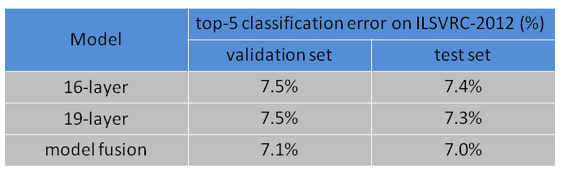# Fcn using pretrained vgg16 in model zoo?

Does anyone tried to implement FCN using the pretrained vgg16 in model zoo?
I tried and tested on SiftFlow dataset but it can’t achieve the same level of performance of original caffe version or my theano version. Below is the detailed codes how I implemented it. Any suggestions will be welcome!In summary, I first implemented a FCN-32 network class and loaded/reshaped pre-trained weights in the same way as caffe did. Before feeding data into the network, every image is scaled to [0 1], subtracted by mean [0.485, 0.456, 0.406], and divided by std [0.229, 0.224, 0.225]. I guess this is the same normalization used for training the model zoo vgg16 network. And the network is trained using the exact same parameters as caffe version.

``````#-------- main part --------
model = FCN_32()

layer_names = [layer_name for layer_name in pre_trained_weights]

counter = 0
for p in model.parameters():
if counter < 26:  # conv1_1 to pool5
p.data = pre_trained_weights[ layer_names[counter] ]
elif counter == 26:  # fc6 weight
p.data = pre_trained_weights[ layer_names[counter] ].view(4096, 512, 7, 7)
elif counter == 27:  # fc6 bias
p.data = pre_trained_weights[ layer_names[counter] ]
elif counter == 28:  # fc7 weight
p.data = pre_trained_weights[ layer_names[counter] ].view(4096, 4096, 1, 1)
elif counter == 31:   # upscore layer
m, k, h, w = 34, 34, 64, 64
filter = upsample_filt(h)
filter = torch.from_numpy(filter.astype('float32'))
p.data = filter.repeat( m, k, 1, 1 )
counter += 1

def upsample_filt(size):
"""
Make a 2D bilinear kernel suitable for upsampling of the given (h, w) size.
"""
factor = (size + 1) // 2
if size % 2 == 1:
center = factor - 1
else:
center = factor - 0.5
og = np.ogrid[:size, :size]
return (1 - abs(og - center) / factor) * \
(1 - abs(og - center) / factor)

#-------- FCN-32 implementation part --------
import torch
import torch.nn as nn
import math
import torch.utils.model_zoo as model_zoo

return nn.Conv2d(in_planes, out_planes, kernel_size=(3,3), stride=(stride,stride),

def conv1x1(in_planes, out_planes, stride=1):
return nn.Conv2d(in_planes, out_planes, kernel_size=1, stride=stride,

class FCN_32(nn.Module):
def __init__(self):
super(FCN_32, self).__init__()

# vgg part
self.conv1_1 = conv3x3(3, 64, stride=1, padding=100)
self.conv1_2 = conv3x3(64, 64)

self.conv2_1 = conv3x3(64, 128)
self.conv2_2 = conv3x3(128, 128)

self.conv3_1 = conv3x3(128, 256)
self.conv3_2 = conv3x3(256, 256)
self.conv3_3 = conv3x3(256, 256)

self.conv4_1 = conv3x3(256, 512)
self.conv4_2 = conv3x3(512, 512)
self.conv4_3 = conv3x3(512, 512)

self.conv5_1 = conv3x3(512, 512)
self.conv5_2 = conv3x3(512, 512)
self.conv5_3 = conv3x3(512, 512)

self.fc6 = nn.Conv2d(512, 4096, kernel_size=7, stride=1, padding=0)
self.dropout = nn.Dropout()
self.fc7 = nn.Conv2d(4096, 4096, kernel_size=1, stride=1, padding=0)

self.score_fr_sem = nn.Conv2d(4096, 34, kernel_size=1, stride=1, padding=0, bias=False)

self.pool = nn.MaxPool2d(kernel_size=2, stride=2, ceil_mode=True)

self.relu = nn.ReLU(inplace=True)

self.softmax = nn.Softmax()

self._initialize_weights()

def forward(self, x):
# vgg part
conv1_1 =  self.relu(  self.conv1_1(x) )
conv1_2 =  self.relu(  self.conv1_2(conv1_1) )
pool1 = self.pool(conv1_2)

conv2_1 =  self.relu(   self.conv2_1(pool1) )
conv2_2 =  self.relu(   self.conv2_2(conv2_1) )
pool2 = self.pool(conv2_2)

conv3_1 =  self.relu(   self.conv3_1(pool2) )
conv3_2 =  self.relu(   self.conv3_2(conv3_1) )
conv3_3 =  self.relu(   self.conv3_3(conv3_2) )
pool3 = self.pool(conv3_3)

conv4_1 =  self.relu(   self.conv4_1(pool3) )
conv4_2 =  self.relu(   self.conv4_2(conv4_1) )
conv4_3 =  self.relu(   self.conv4_3(conv4_2) )
pool4 = self.pool(conv4_3)

conv5_1 =  self.relu(   self.conv5_1(pool4) )
conv5_2 =  self.relu(   self.conv5_2(conv5_1) )
conv5_3 =  self.relu(   self.conv5_3(conv5_2) )
pool5 = self.pool(conv5_3)

fc6 = self.dropout( self.relu(   self.fc6(pool5) ) )
fc7 = self.dropout( self.relu(   self.fc7(fc6) ) )

score_fr_sem = self.score_fr_sem( fc7 )

upscore_sem = self.upscore_sem(score_fr_sem)

crop = upscore_sem[:, :, 19:19+256, 19:19+256] # batch, 34, 256, 256

crop = crop.transpose( 1, 3 )
crop = crop.transpose( 1, 2 ) # batch, 256, 256, 34

output = crop.contiguous().view(-1, crop.size(3))

output = self.softmax(output)

return output

def _initialize_weights(self):
for m in self.modules():
if isinstance(m, nn.Conv2d):
n = m.kernel_size * m.kernel_size * m.out_channels
m.weight.data.normal_(0, math.sqrt(2. / n))
if m.bias is not None:
m.bias.data.zero_()
elif isinstance(m, nn.BatchNorm2d):
m.weight.data.fill_(1)
m.bias.data.zero_()
elif isinstance(m, nn.Linear):
n = m.weight.size(1)
m.weight.data.normal_(0, 0.01)
m.bias.data.zero_()``````

I guess, maybe the performance of this pretrained vgg16 model is worse than the original oxford vgg16 model.
You can try to convert the original vgg16 model into pytorch format, and change the preprocessing step accordingly.

@shicai Yes, I think you are right. I tried the vgg model converted by Justin and it gave better performance.

Any idea why it’s worse?

@ecolss it is hard to clearly figure out why it is worse, but I personally think it is due to the lack of data augmentation and pretraining.

So you mean, the pretrained vgg model by pytorch is not done exactly the same way as vgg paper did, right?

No, it is not. Tt least provided vgg model used a different image preprocess method.

Well, if that’s the case, then if you follow the exactly same preprocessing method which trained the pytorch model, then you would get the same accuracy as you would get on a caffe converted model, isn’t it?

@ecolss maybe.
at this time, it is not very difficult to reproduce the performance of vgg models. just need some time.

Yes, you are right. The performance is lower than expected even using the same preprocessing method. Using caffe converted model is better.

Sam has updated the models now with more accurate models.

Previous | New error rates

``````-VGG-11      32.14           12.12	 +VGG-11       30.98           11.37
-VGG-13      31.04           11.40	 +VGG-13       30.07           10.75
-VGG-16      29.11           10.17	 +VGG-16       28.41            9.62
-VGG-19      28.42            9.69	 +VGG-19       27.62            9.12
``````

@smth should we put batchnorm layers in linear classifier layers for vgg_bn models?
nn.Linear is actually 1x1 nn.Conv2d.

vgg does not have BatchNorm

by using batchnorm layers during training, we can get better performance for vgg models.

i understand, but we cannot put it into the reference VGG model, everyone expects VGG to be of a certain architecture that mirrors the paper.

1 Like

WELL, previous error rate is so intimidating.

Isn’t this error rate still far off the original error rate? So are the pytorch vgg models about 2% worse than the original caffe vgg?